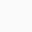# Molarity of Glacial Acetic Acid (99.7%, w/w, CH3COOH)

• Glacial acetic acid is a clear colorless liquid. A 99.7% (w/w) concentrated glacial acetic acid can be obtained from different suppliers.
• A 99.7% (w/w) glacial acetic acid means that 100 g of glacial acetic acid contains 99.7 g of acetic acid.
• The density of glacial acetic acid is 1.049 g/ml at 25°C which means that the weight of the 1 ml of glacial acetic acid is 1.049 grams at 25°C.
• Molarity refers to the number of moles of the solute present in 1 liter of solution.
• In simple words, 1 mole is equal to the atomic weight of the substance. For example, 1 mole of acetic acid is equivalent to 60.05 g of acetic acid (molecular weight = 60.05).

## Calculation procedure:

Step 1: Calculate the volume of 100 grams of glacial acetic acid solution.
Formula:

or

Note: 99.7% (w/w) Glacial acetic acid means 100 g of glacial acetic acid contains 99.7 g of acetic acid.

The volume of 100 g of glacial acetic acid is 95.329 ml. That means 99.7 grams of acetic acid is present in 95.329 ml of glacial acetic acid.

Step 2: Calculate how many grams of acetic acid is present in 1000 ml of glacial acetic acid.
95.32 ml of glacial acetic acid contains = 99.7 grams of acetic acid

1000 ml of glacial acetic acid will contain 1045.85 grams of acetic acid.

Step 3: Calculate the number of moles of acetic acid present in 1045.85 grams of acetic acid.
60.05 grams of acetic acid = 1 mole

moles

Therefore, we can say that 1 liter of glacial acetic acid contains 17.416 moles or in other words molarity of glacial acetic acid (99.7% w/w, density 1.049 g/ml) is equal to 17.416.Use Calculator to calculate the Molarity of concentrated acetic acid (CH3COOH) when concentration is given in % by mass (w/w)

The molecular weight of Acetic acid (CH3COOH): 60.05 g/mol

Concentration of acetic acid : % (wt/wt)

(Change the % (wt/wt) concentration)

Density of glacial acetic acid: g/ml

(Change the density)

Molarity of acetic acid: 17.416 M1.Sagar Joshi says: